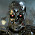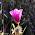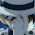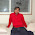## Thursday, August 20, 2009

### C++: Reference to Pointer

Recently working with some code, I came across an interesting concept - references to pointers. What does that even mean? Why would you need a reference to a pointer - ever?

After spending some time with my friend Google, I found out the answer. Suppose we have these two function definitions, and a main function:
```void function_a(int *& a) {
*a += 5;
int * c = new int(7);
a = c;
}```
```void function_b(int * a) {
*a += 5;
int * c = new int(7);
a = c;
}
```
```int main() {
int * myInt = new int(5);
int * myInt2 = new int(5);

function_a(myInt);
// what is the value of myInt?

function_b(myInt2);
// what is the value of myInt2?

return 0;
}```

Analysis

Above, in 'function_a' we're actually passing in 'int *& a', which is a 'reference to a pointer' - a better way to think of this as passing in a 'pointer by reference'. Now, I admit, when I first saw this I thought it was called a 'pointer to a reference' but I'm not really sure what that actually would mean. So for now, just take my word for it that it's called a reference to a pointer. :)

Ok, well if you look at 'function_b', it's passing in it's paramter by pointer only ('int * a'). Both functions do the same operations within their bodies, so whats with the ampersand in function_a? Lets assume that you compiled this application and ran it. Using the int main above, you would expect the value of myInt to be 7 after function_a, right? How about for myInt2? Again, you'd expect it to be 7 after function_b. That's where you're wrong though. After running the main function above you'll get this output:

• myInt = 7
• myInt2 = 10

Hooray for unexpected results! The reasoning behind this actually makes sense when you realize this rule for C++:

All parameters are passed by value, unless the ampersand is specified.

Yes, even pointers are passed by value, meaning that a local copy is made for that function. This gets confusing because a pointer simply stores a memory address - and you can change the value at that memory address. The key though, is that if you make a local copy of that memory address in another variable, you can still modify the area in memory that memory address refers to, but now since it's a copy of that, if you try to change the address, it only changes for the scope the variable lives in. Confused? Lets see an example:

int * outside - points to memory at 0xFF9999, and lets say the value at that address is the integer '27'.

suppose we write

```int * outside = new int(27);
function_b(outside);  // Using function_b from above
```
1. Inside function_b, our local int pointer 'a' now has a copy of that memory address 0xFF9999.
2. We first add 5 to the value at that address the way we normally would... So, 27+5 = 32. Now 'a' has a value of 32. And so does 'outside', since they both point to the same location. This is what we expected!
3. Now we initialize int * c = new int(7) - so 'c' has a value of 7 and lets say it has an address of 0x22CCCC.
4. Then we set the address of 'a' to 'c'. Well 'a' now has the address 0x22CCCC, and consequently the value 7, but we're no longer changing the 'outside' pointer value, since 'a' was a copy of 0xFF9999. So 'outside' still is pointing to 0xFF9999, and 'a' is pointing to '0x22CCCC'.
5. The function returns, 'outside' now has a value of 32, and the memory at address 0x22CCCC has been leaked since nothing is pointing to it any longer!

How Passing a Pointer by Reference Solves this Problem

When we pass the pointer by reference, as in function_a, the local variable 'a' is no longer a copy of the memory address that 'outside' points to, it literally is the variable 'outside'. Therefore setting the address of 'a' to 'c', will actually change the value of 'outside' to 7, as it changes the address of 'outside'. (You may have noticed that function_a also leaks memory as it never deletes the memory that 'a' pointed to before switching to 'c' as well!)

Hopefully this explanation ends up helping someone out there that may encounter this problem, which seems to be some what not well documented and untaught in basic programming classes in school!

Feel free to post about your experiences with references to pointers below.
Thanks!

1.Hi Mark,

I would like to suggest to present compilable code, which reflects the presented semantics (especially 'a') :).

void function_a(int *& a)
{
*a += 5;
int * c = new int(7);
a = c;
}

void function_b(int * a)
{
*a += 5;
int * c = new int(7);
a = c;
}

int main()
{
int * myInt = new int(5);
int * myInt2 = new int(5);

function_a(myInt);
// what is the value of myInt?

function_b(myInt2);
// what is the value of myInt2?

return 0;
}

2.Thanks so much for checking it! :) I've updated the above code to reflect your changes.

3.Very nice tutorial. It helps me to understand when to use & especially when the parameters are pointers. Thank you!

4.Yeah dude - the same goes for memory management. If you have a class that holds a huge array on the heap, and then you have a utility function to delete this array, make sure you have the parameter be a reference to the actual array.

Otherwise, C++ never deletes the right memory.

5.Thanks for your post.... I learnt a lot with your simple & concise explanation. I was having a really hard time understanding why my code wasn't working.

I will go forth & conquer C++ now :)

Asif

6.Awesome!! Thanks a bunch! Really helped. This stuff is definitely not very well documented. :)

7.Thanks a lot, Mark! It helps me understand reference much better!

8.Thank you for a concise, well-written explanation.

It is amusing to compare this article to the MSDN entry on the same topic: http://msdn.microsoft.com/en-us/library/1sf8shae.aspx

I think I had a small stroke while trying to quickly parse Microsoft's example.

9.you should write a book...with hundreds of these amazing 'step-by-step' atricles.

....
..

yeah...

10.Many Thanks!
Your elucidation is much clearer and easier to understand than MSDN. ^-^

11.Thanks for your help !

12.results expected but why 7 + 5 = 10? it should be 12.

7 after first function + 5 after second one.

13.Thanks for exposing such an interesting cogito

14.Yups :) you explained it pretty well. I was banging my head against the wall on a similar problem. Your article explains it pretty well. Keep it up

15.There is one more useful application of references to pointers. It is a well know idiom to use wrapper functions for static data: it solves the problem of "static initialization order fiasco". So if you have a static pointer you want to wrap into function, reference to pointer is what this function should return.

16.Great article. I have a question though, hopefully someone can explain this last bit:
" (You may have noticed that function_a also leaks memory as it never deletes the memory that 'a' pointed to before switching to 'c' as well!)"

How would one go about solving this problem?

1.The first thing i would try is to just call Delete on the 'a' pointer before assigning it the address of 'c'. The var 'a' actually 'is' myInt at this point, so i would expect to call "delete(a)" which should erase the contents pointed to by 'a' (also myInt), before it is assigned to point to a new int on the heap ('c'). Also you would do the same for the other function as this would reference the local copy of 'a'.

As 'a' is the same as myInt, the dereference is required to increase the value by 5 and not the address its currently pointed to.

Rich.

2.The first thing i would try is to just call Delete on the 'a' pointer before assigning it the address of 'c'. The var 'a' actually 'is' myInt at this point, so i would expect to call "delete(a)" which should erase the contents pointed to by 'a' (also myInt), before it is assigned to point to a new int on the heap ('c'). Also you would do the same for the other function as this would reference the local copy of 'a'.

As 'a' is the same as myInt, the dereference is required to increase the value by 5 and not the address its currently pointed to.

Rich.

3.... or, in the off chance that your function doesn't *need* to change the 'a' pointer, you can avoid mistakenly introducing a memory leak by using const in the protocol. Like:

void function_a(const int *& a)

Then the compiler won't let you change what 'a' points to. ('c' could still lead to a leak though.)

17.Thanks! it solved my problem

18.Hi Mark, it was a good explanation. still i have a doubt.
In the function using reference, you have created one more new varibale and assigned that address to variable a(outside) now both a and outside points to the same memory address.But what about the memory which was already allocated in main function.

Kindly Clarify me.

19.Although I read a lot about this before, but nothing compares with a simple example with detailed explanation. Thanks!

20.Fantastic !

21.No wonder this is the #1 ranked site for the Google search "c++ reference to pointer"... great explanation!

22.Thank you so much for explaining it so clearly!

23.Thanks so much for taking the time to write this!! I've been searching for a clear explanation on this for a while now :) This really helped!

I realize this was posted a long time ago, but I have another question. If you were writing this code in C, would the function b work differently, i.e. in C are pointers passed by reference or value?

24.Excellent explanation!
Going to check out your blog for more gems.

25.Your blog is awesome. Good job. Pointers are without a doubt one of the most important—and troublesome—aspects of C++. In fact, a large measure of C++’s power is derived from pointers. For example, they allow C++ to support such things as linked lists and dynamic memory allocation. They also provide one means by which a function can alter the contents of an argument. However, these and other uses of pointers will be discussed in subsequent chapters. In this chapter, you will learn the basics about pointers, see how to manipulate them, and discover how to avoid some potential troubles. In a few places in this chapter, it is necessary to refer to the size of several of C++’s basic data types. For the sake of discussion, assume that characters are one byte in length, integers are four bytes long, floats are four bytes long, and doubles have a length of eight bytes. Thus, we will be assuming a typical 32-bit environment.

26.This is exactly what I am looking for ..thank you mark

27.I think the following example demonstrates how to use reference-to-ptr, if you want to swap pointers :

#include

using namespace std ;

void swapval ( int val1, int val2 )
{
int temp = val1 ;
val1 = val2 ;
val2 = temp ;
}

void swapptr ( int* val1, int* val2 )
{
int temp = *val1 ;
*val1 = *val2 ;
*val2 = temp ;
}

void swapref ( int& val1, int& val2 )
{
int temp = val1 ;
val1 = val2 ;
val2 = temp ;
}

void swaprefptr ( int*& val1, int*& val2 )
{
int* temp = val1 ;
val1 = val2 ;
val2 = temp ;
}

//
// This is the code demonstrating different approaches of swapping
// values
//
// Otar M. Davitashvili
// otar_david@hotmail.com
//

int main ()
{
int *val1 = new int (100),
*val2 = new int (200) ;

std::cout << "Before swapval v1 = " << val1
<< " *v1 = " << *val1 << " v2 = " << val2
<< " *v2 = " << *val2 << '\n' ;

swapval ( *val1, *val2 ) ;

std::cout << "After swapval v1 = " << val1
<< " *v1 = " << *val1 << " v2 = " << val2
<< " *v2 = " << *val2 << '\n' ;

swapptr ( val1, val2 ) ;

std::cout << "After swapptr v1 = " << val1
<< " *v1 = " << *val1 << " v2 = " << val2
<< " *v2 = " << *val2 << '\n' ;

swapref ( *val1, *val2 ) ;

std::cout << "After swapref v1 = " << val1
<< " *v1 = " << *val1 << " v2 = " << val2
<< " *v2 = " << *val2 << '\n' ;

swaprefptr ( val1, val2 ) ;

std::cout << "After swaprefptr v1 = " << val1
<< " *v1 = " << *val1 << " v2 = " << val2
<< " *v2 = " << *val2 << '\n' ;

delete val1, val2 ;
}

28.Great explanation. You will encounter references to pointers in binary (and other) tree operations. When inserting new nodes, you have to pass a pointer by reference to ensure the actual node at the insertion point points to the new node, as opposed to a copy of the node pointing to the new node.

29.its amazing.i love this explanantion

30.Thanks! This fix is very interesting and an eye opener!

31.Wonderful post. Thanks

32.Thanks!... great tutorial!

33.Awesome today I encountered it for the first time and learntit as well. thanks a bunch.

34.i found this article which goes on to explain Pointers to Pointers as well.

35.Very cool article!

36.Good explanations!

37.Actually a nice article ,I've always wanted to know the difference between references and pointers variables in C++ .

Regards
Jacky

38.Fantastic indeed!

39.Great article and explanation, thank you

40.your post is really very helpful!!! THanks ... :-)

41.Thanks a lot!